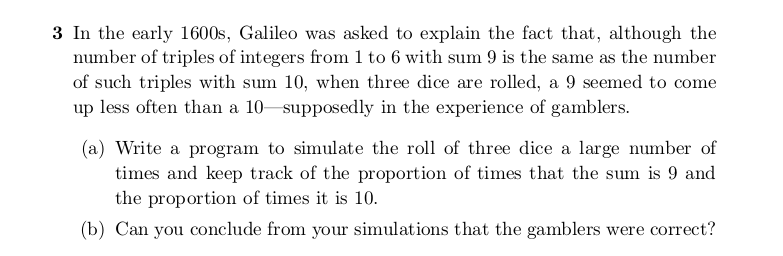### sample to sample data and keep track of occurences the sum of 3 dice were 9 and 10

# number of times to run this simulation of 10000 trials/runs
n_sim <- 100 # 100 times simulate 10000 trials

total_times <- rep(FALSE, n_sim) # vector to store all simulation results

for (i in 1:n_sim){

sum_9 <- 0 # counter for # of times sum == 9
sum_10 <- 0 # counter for # of times sum == 10
n <- 10000 # number of times to roll 3 dice

# for loop to simulate
for (j in 1:n){
dice <- sample(1:6, 3, replace = TRUE) # pick a number from 1 to 6 3 times with equal likelihood
if (sum(dice) == 9){ # if the sum of the rolled 3 dice is 9
sum_9 <- sum_9 + 1 # increment the counter
}
if (sum(dice) == 10){ # if the sum of the rolled 3 dice is 10
sum_10 <- sum_10 + 1
}
}

# check if the # of sum == 9 is less than # of sum == 10
if (sum_9 < sum_10){
total_times[i] <- TRUE # mark as true
}
}

### Running this simulation 100 times of rolling 3 dice 10000 times:

print(sum(total_times == TRUE))
##  97